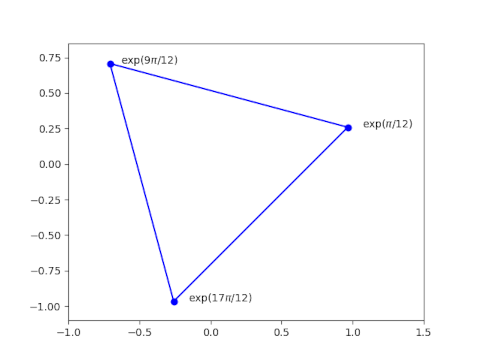# Test whether three complex numbers are vertices of an equilateral triangle

Let a, b, and c be three complex numbers.These numbers form the vertices of an equilateral triangle in the complex plane if and only ifThis theorem can be found in .

If we rotate the matrix above, we multiply its sign by -1. If we then swap two rows we multiply the determinant again by -1. So we could write the criterion above with the 1’s on the top row.See also this post which gives the area of a triangle in the complex plane, also in terms of a determinant.

 Richard Deaux. Introduction to the Geometry of Complex Numbers.

## One thought on “Test whether three complex numbers are vertices of an equilateral triangle”

1. Andrew

-x only equals 0 if x equals 0 :)